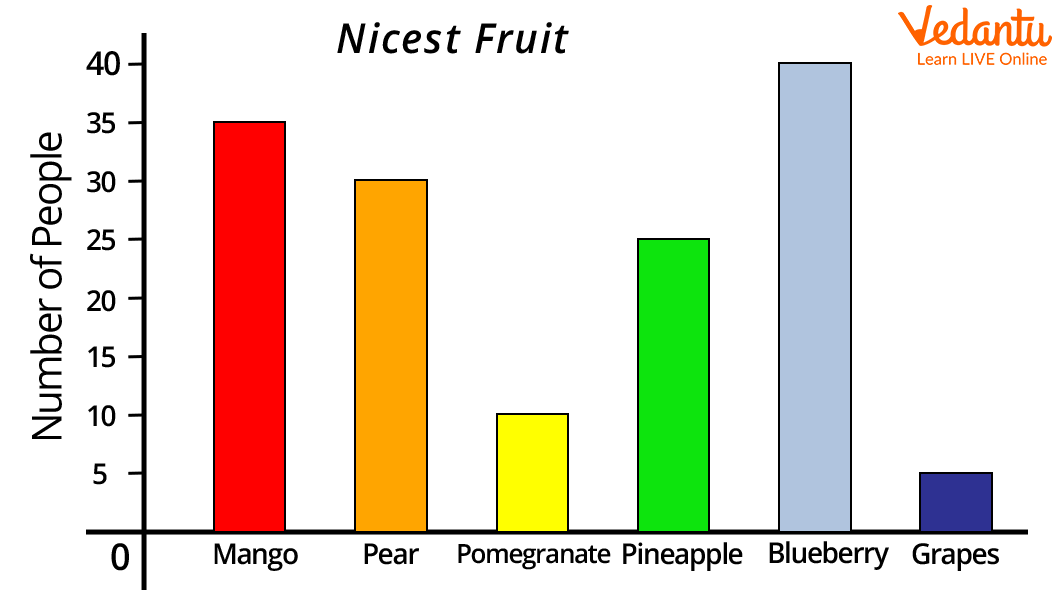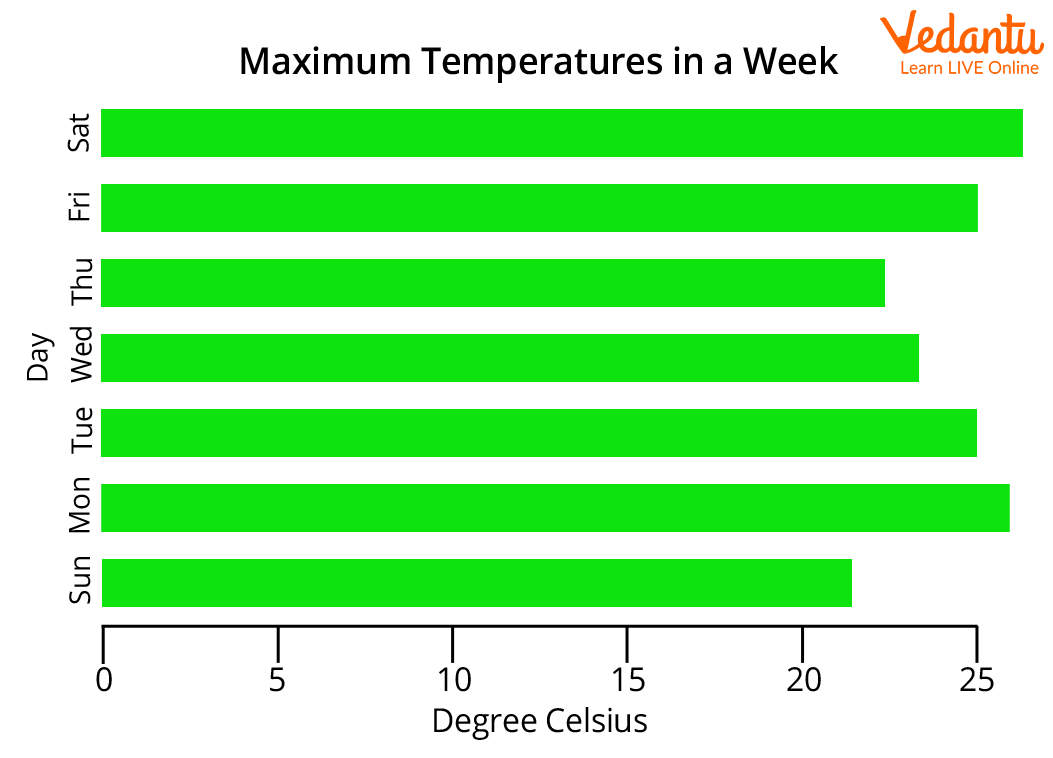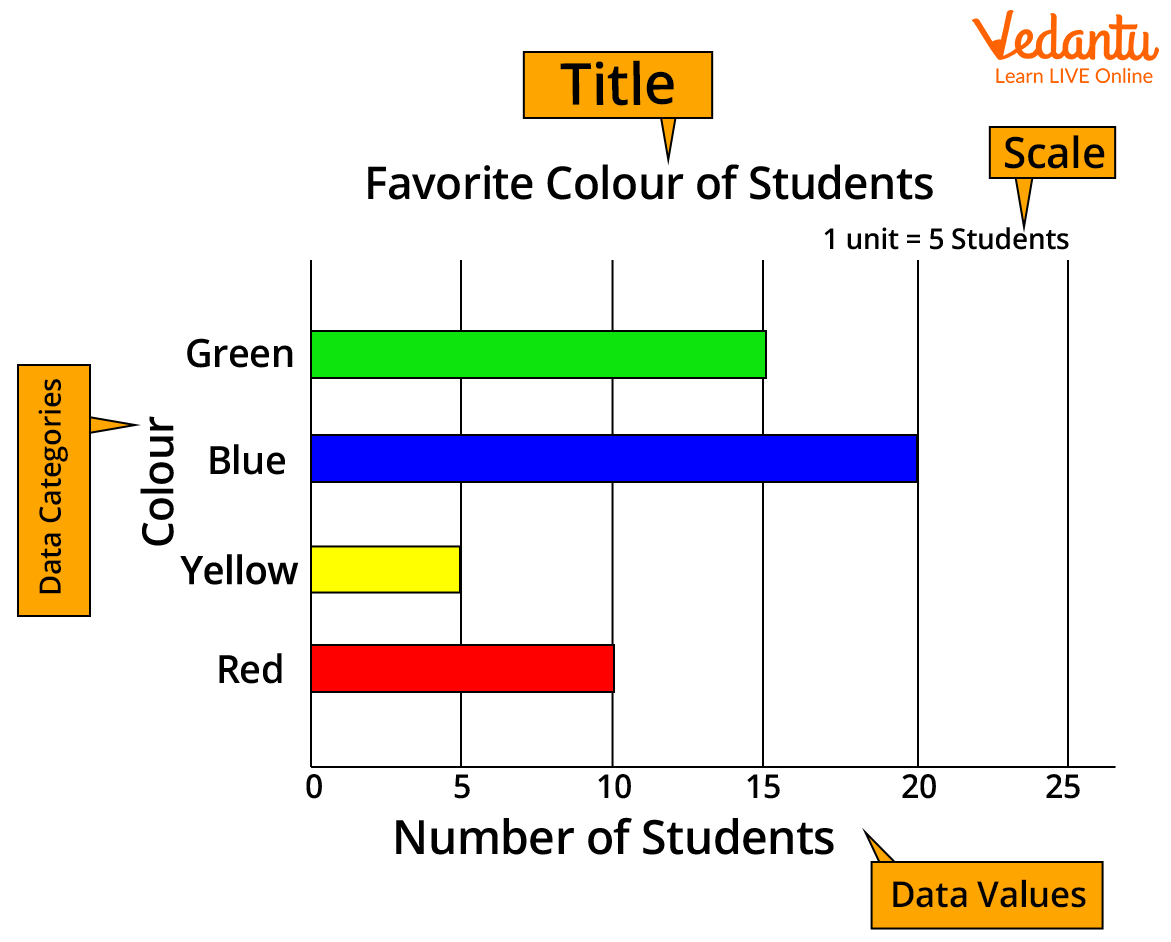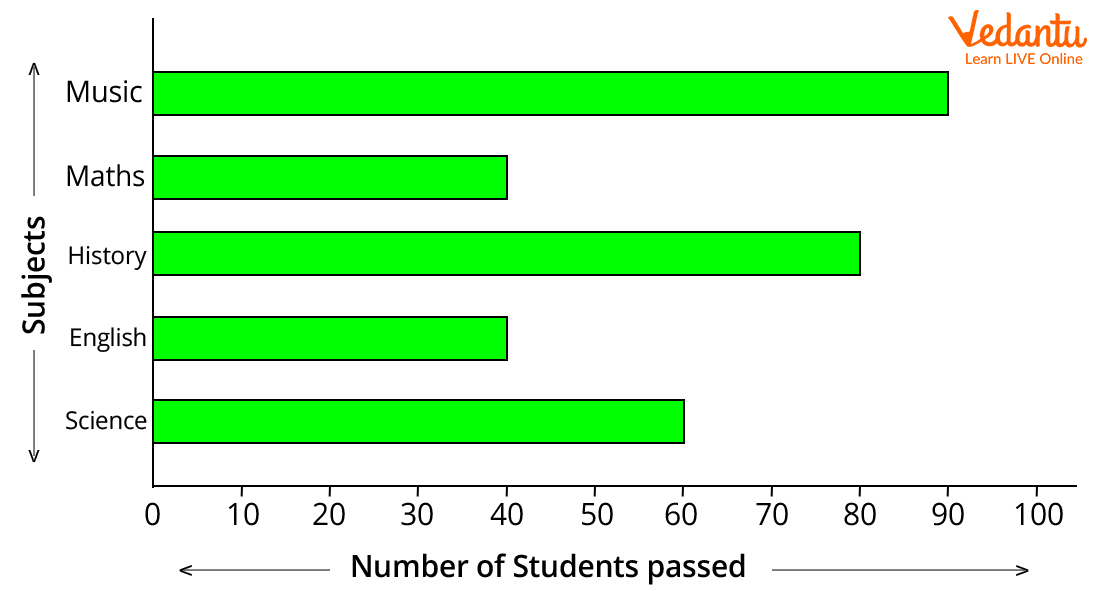Courses
Courses for Kids
Free study material
Offline Centres
More

# Bar Graph Horizontal: How to Construct Horizontal Bar?Last updated date: 01st Dec 2023
Total views: 158.1k
Views today: 4.58k## Horizontal Bar Graph

Have you heard the term Bar graph horizontal or horizontal bar graph? A horizontal bar graph or bar graph horizontal is nothing but a way to represent data horizontally in a graph using bars. In horizontal bar graphs, we represent data categories on the x-axis whereas data values are on the y-axis. Horizontal bar graphs are generally used to compare different observations. Read the article below to have detailed information on Bar Graph Horizontal with interesting examples.

## What is a Bar Graph?

Let us first know what a bar graph is, before knowing the horizontal bar graph in detail.

A bar graph, also known as a bar chart, is a graphical representation of data using bars of different heights.

Imagine you did a survey of 145 people to know which type of fruits are most liked by people.

 Fruits Apple Oranges Banana Kiwifruit Blueberries Grapes People 35 30 10 25 40 5

On the bar graph, we can show this information as:Image: Bar graph of fruits

A bar graph is an optimum way to show relative size. For example, in the above bar graph, we can say blueberries are most liked by the people whereas grapes are least liked by the people.

## Types of Bar Graph

There are two different types of bar graphs namely:

• Horizontal bar graph and

• Vertical bar graph

## What Does Horizontal Bar Graph Mean?

A horizontal bar graph or bar graph horizontal is a way to represent data horizontally in a graph using bars. In horizontal bar graphs, data categories are represented on the x-axis whereas data values are represented on the y-axis. Horizontal bar graphs are widely used for easy and quick comparison among various observations based on certain parameters. In horizontal bar graphs, the length of the rectangular bars is proportional to their values. Also, all bars in the horizontal bar graph go from left to right.

## How to Construct a Horizontal Bar Graph?

Following are the steps to construct a horizontal bar graph:

Step 1: On a graph paper, draw two perpendicular lines intersecting at O.

Step 2: The horizontal line on the bar graph is the x-axis whereas the vertical line on the bar graph is the y-axis.

Step 3: Along a horizontal axis, choose a suitable scale in order to determine the height of the bar for the given values (frequency is represented along the y-axis).

Step 4: Along a vertical axis, choose the uniform width of the bar, and uniform gap between the bars. Also, write the name of the data items whose values are to be marked.

Step 5: Calculate the height of the bar according to the value chosen for each graph and draw the horizontal bars accordingly.

Step 6: Give a suitable title to the graph.

The horizontal bar graph given below gives the information about temperature in celsius on different days of the week.Image: Horizontal bar graph showing maximum temperature in a week

## How to Read a Horizontal Bar Graph?

The horizontal bar graph is ead in the following manner:

• The title of the bar graph tells about the data being represented by the graph.

• The vertical axis of the horizontal bar graph represents the data categories. The data categories in the horizontal bar graph given below are “colours”.

• The horizontal axis of the horizontal bar graph represents the values corresponding to each data value. The data values in the below horizontal bar graph represent the number of students who like a particular colour represented on the vertical axis.

• The scale is showing the value of 1 unit on the horizontal axis.From the above horizontal bar graph, we can infer that 1 unit represents 5 students. Accordingly, 10 students like the red colour, 5 students like the yellow colour, 20 students like blue colour, and 15 students like the green colour.

## Horizontal Bar Graph Example

Let us understand the horizontal bar graph with an example:

Draw a horizontal bar graph on the basis of the following data.

 Subjects Music Maths History English Science Number of Students Passed 90 40 80 40 60Image: A bar graph showing subjects and the number of students who passed

The horizontal bar graph gives the following information:

• The total number of students passed in different subjects.

• The maximum number of students passed in Music.

• The minimum number of students passed in English and Maths.

• Students scored highest marks in Music but scored worst in English and Maths.

Hope you have understood what a horizontal bar graph is, and how to read and represent a horizontal bar graph. Now, you can visit Vedantu’s official website and try different questions based on this to understand the concept better.

## FAQs on Bar Graph Horizontal: How to Construct Horizontal Bar?

1. When is Horizontal Bar Graph Mostly Preferred?

The horizontal bar graph is a preferred option when larger quantities of data are involved as the positioning of labels on the vertical axis offers better readability.

2. What values are mostly represented in horizontal bar graphs?

Ensure to create a horizontal bar graph to represent a value that is nominal/categorical. The example of nominal data includes the city of residence, blood type, political party, favourite food, etc. Also discussed above, horizontal bar graphs are ideal for larger data sets or those with longer labels.

3. What does the title of the horizontal bar graph represent?

The tile of the horizontal bar graph provides precise information on what is there in your graph. This enables readers to know what they are about to look at. The title of the bar graph should be creative and easy to read.